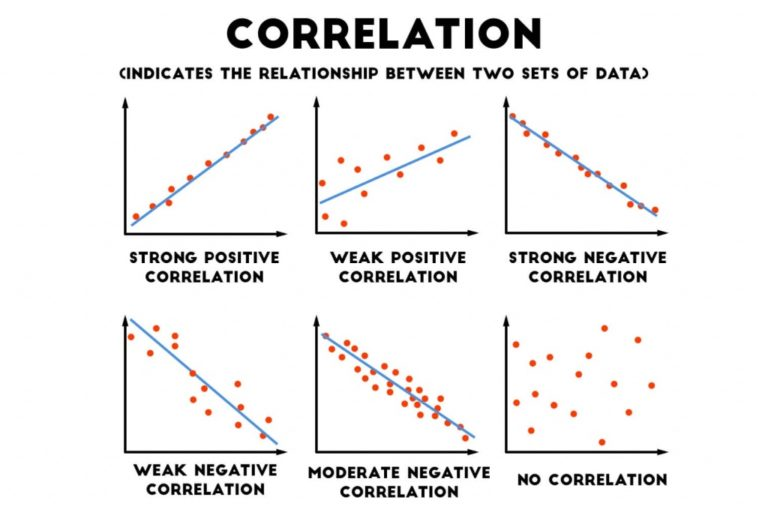# Correlation in a Whole: Negative & Positive Correlation, Examples

The compilation of these Correlation Notes makes students exam preparation simpler and organised.

## Correlation in a Whole

Correlation in a Whole
We know that when the price of a product increases its demand will decrease. And to the contrary quality supplied will increase with the increase of price. These are nothing but positive correlations and negative correlations. Statistical correlation, its meaning, and types and examples are defined in this lesson.

### Correlation: Definition and Types

Correlation can be defined as a statistical tool that defines the relationship between two variables. For, eg: correlation may be used to define the relationship between the price of a good and its quantity demanded. It explains how two variables are related but do not explain any cause-effect relation. It only gives an understanding as to the direction and intensity of the relation between two variables. Correlation can be of two types:

1. Positive Correlation:
Two variables are positively correlated when they move together in the same direction. In economics, quantity supplied increases as the price increases. This is because sellers find it profitable to sell when the prices are high, so they will sell more. Thus, we can call price and quantity supplied to be positively correlated. This is also called the law of supply.

2. Negative Correlation:
Two variables are negatively correlated if they move in opposite directions. For instance, as the price of increases, the quantity demanded declines as the good becomes more expensive relative to when the price had not increased. Thus, we can say that price and quantity demanded are negatively correlated. Note that this is the famous law of demand.

### Correlation Estimation

There are different ways of calculating or estimating the correlation between variables.

1. Scatter Diagram
This is a diagrammatic method of correlation estimation. Here, the x-values are depicted on the horizontal axis and y-values are on the vertical axis. The degree of closeness between the coordinates would indicate the correlation. If the trend is downward sloping from right to left, it means a negative correlation.

If it is upward sloping from left to right, it is upward sloping. And if the points are scattered all around with no trend, then the variables are said to be uncorrelated.2. Karl Pearson’s Correlation Coefficient
This is a quantitative method of correlation estimation. The formula is:
r = cov(x, y)/σx . σy
where, cov(x, y) is the covariation between the two variables. And, σx and σy are the standard deviations of x and y variables, respectively. Properties of ‘r’:

• It has no unit.
• It lies between -1 and +1, both included. In both the extreme cases, there is either a perfect negative or perfect positive correlation, respectively.
• A value of zero means no correlation.
• A high value of ‘r’ indicates a strong linear relationship, and vice versa.
• A positive value indicates a positive correlation.
• The value of ‘r’ is unaffected by a change of origin or change of scale.

3. Spearman’s Rank Correlation
This method is a non-parametric method of correlation estimation. It is used to determine the correlation between the ranks of different variables. These variables are usually qualitative in nature, such as beauty, honesty, intelligence, wisdom, etc. Ranks such as first, second, third, etc. are assigned and then the correlation is calculated using a formula.

Degree of Correlation

• +1 – Perfect Positive
• -1 – Perfect Negative
• 0 – Uncorrelated

In other cases (positive or negative), if the value of ‘r’ is 0.50, it is called a moderate correlation. When it lies between 0.50 and 0.75, the degree of correlation is high and when it lies between 0.25 and 0.50, the degree of correlation is low.

Example:

Question:
Can the value of ‘r’ lie outside the range of -1 to 1?